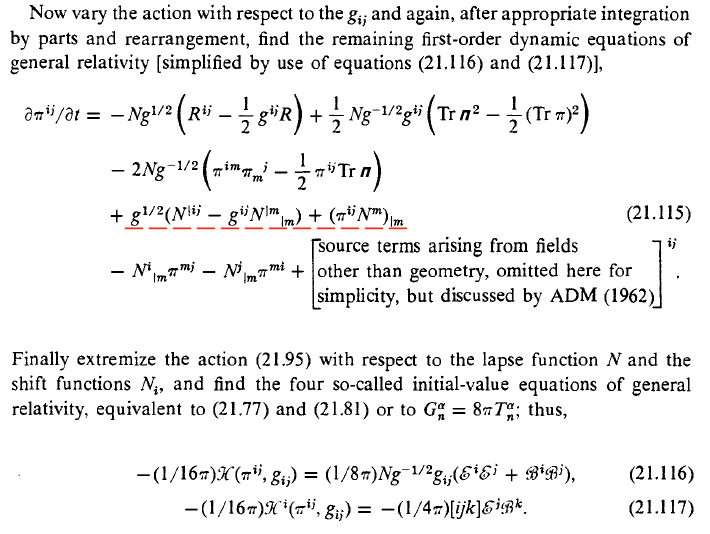# Initial Value Equations out of ADM formalism

Gold Member
Homework Statement:
Use the ADM formalism to derive dynamic and initial value equations
Relevant Equations:
MTW (21.115) - See attachment
In my earlier post, I demonstrated a way to derive MTW's Equation (21.90),

##16π\mathfrak{L}_{geom} = - \dot π^{ij} γ_{ij} - N\mathcal{H} -N_i\mathcal{H^i}-2(π^{ij}N_j-½N^iTrπ+γ^½N^{|i})_{,i}## MTW (21.90)

I received my first two 'likes' for this which made me really happy - Thanks guys!

I've moved on a bit now and have reached page 525, (four whole pages - wow!) and I am now trying to produce MTW (21.115) - see attachment.

MTW suggests that this can be derived from:

##I = \frac{1}{16π}\int[ \dot π^{ij} γ_{ij} - N\mathcal{H} -N_i\mathcal{H^i}]d^4x + \int\mathfrak{L}_{Field}d^4x ## MTW (21.95)

I've managed to produce nearly all of (21.115) but I cannot find a way to produce the terms I've underlined.

Instead of the term ##(π^{ij}N^m)_{|m}## I have ##(N^iπ^{jk})_{|k}##

I can't see any way to produce terms with ##N^{|ij}## or ##N^{|m}{}_{|m}## from any of the terms in 21.95, but if I go back to (21.90), I can use the term ##[N^{|i}(\gamma)^{\frac{1}{2}}]_{,i}##, which nearly gets me home, but I end up with

##(\gamma)^{\frac{1}{2}}N^{|ij} - \frac{1}{2}(\gamma)^{\frac{1}{2}}g^{ij}N^{|m}{}_{|m}##

and a bonus term## -(-g)^{\frac{1}{2}}\frac{1}{N^2}N^{|i}N_{|i}##

(I have a copy of the original ADM paper and interestingly, they do use the full (21.90) to derive (21.115), ie without dropping the divergence!)

Is there something I have missed that would enable me to show that the bonus term somehow resolves my problems, or is there another route altogether?

Regards

Terry W

Attachment: